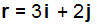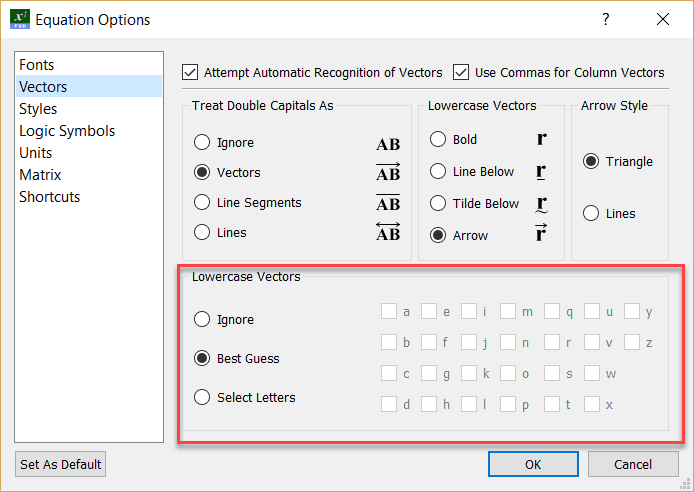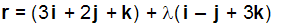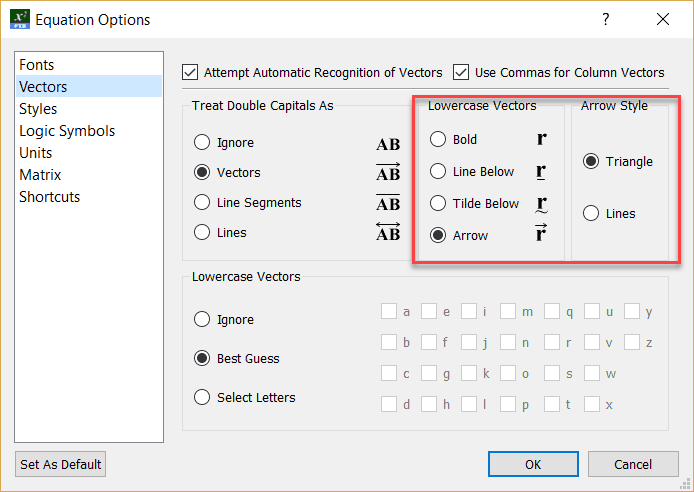﻿ Lowercase Vectors (COPY)

# Lowercase Vectors

Top  Previous  Next

The equation tool will only detect lowercase vectors if automatic recognition is switched on.

If you are using vectors of the formThe equation tool can automatically recognise that you are typing a vector and make the r, i and j bold. The equation tool can also be set to indicate vectors using lines, tildes or arrows, depending on your preference.

This automatic recognition of vectors can save you hours of time, but the equation tool may sometimes get the automatic recognition incorrect.  By default, the equation tool will take its "best guess", you can also turn this feature off or alternatively, explicitly tell the equation tool which variables to recognise as vectors.It is important to remember that you do not need to tell the equation tool that you are working with a vector, the equation tool will automatically identify this.

## Example:is entered as r=(3i+2j+k)+la(i-j+3k)

Lowercase vectors can be display bold, with an arrow over the top or with a line or tilde underneath the vector.